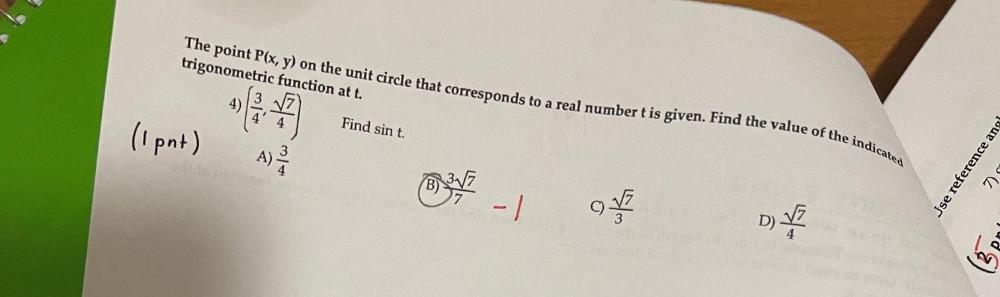Question:

# The point P(x, y) on the unit circle that corresponds to a real number t is given. Find the value of the indicated trigonometric function at t. (3/4, √7/4) Find sin t. A) 3/4 B) 3√7/7 C) √7/3 D)The point P(x, y) on the unit circle that corresponds to a real number t is given. Find the value of the indicated trigonometric function at t. (3/4, √7/4) Find sin t. A) 3/4 B) 3√7/7 C) √7/3 D) √7/4